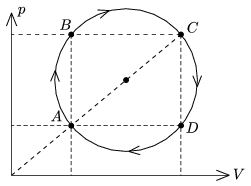Mathematical and Physical Journal
for High Schools
Issued by the MATFUND Foundation
 Already signed up? New to KöMaL?

#Problem P. 4386. (November 2011)

P. 4386. A sample of ideal gas of some mass is taken through the cyclic process shown in the figure. The temperature of the gas at state A is TA=200 K, and at state C is TC=1800 K.a) By what factor is the pressure at state B greater than at state D?

b) What temperature belongs to the isotherm which goes through the centre of the circle?

c) Prove with calculations that the temperatures at state B and D are the same.

(3 pont)

Deadline expired on December 12, 2011.

Sorry, the solution is available only in Hungarian. Google translation

Megoldás. $\displaystyle a)$ $\displaystyle p_{B}=3\cdot p_{D}.$

$\displaystyle b)$ $\displaystyle T_\text{közép}=4\,T_{A}=800$ K.

$\displaystyle c)$ $\displaystyle T_{B}=3\cdot 1 \cdot T_{A}= 1\cdot 3 \cdot T_{A}=T_{D}=600~$K.

### Statistics:

 97 students sent a solution. 3 points: 65 students. 2 points: 13 students. 1 point: 8 students. 0 point: 9 students. Unfair, not evaluated: 2 solutionss.

Problems in Physics of KöMaL, November 2011